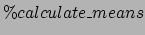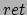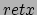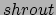Next: Subsetting observations from large Up: Loops Previous: Counters are dates

### %FOR variable in &LIST

I often want to provide a list of variables, say, to a macro and have it run for each of them, instead of cluttering up my code with several invocations of the same macro for each of the variables.

Suppose I want to run the macrofor three variables,,and. I could say:

```  %calculate_means(ret);
%calculate_means(retx);
%calculate_means(shrout);
```

Or I could write a foreach macro:

```  %macro foreach(listofvariables);

%let i=1;
%do %while(%scan(&listofvariables, &i, ' ')>0);
%let thevar=%scan(&listofvariables, &i, ' ');

%put We have the variable &thevar;

%calculate_means(&thevar);

%let i=%eval(&i+1);
%end;
%mend;
```

And call it with

```%foreach(ret retx shrout);
```

Andre de Souza 2012-11-19